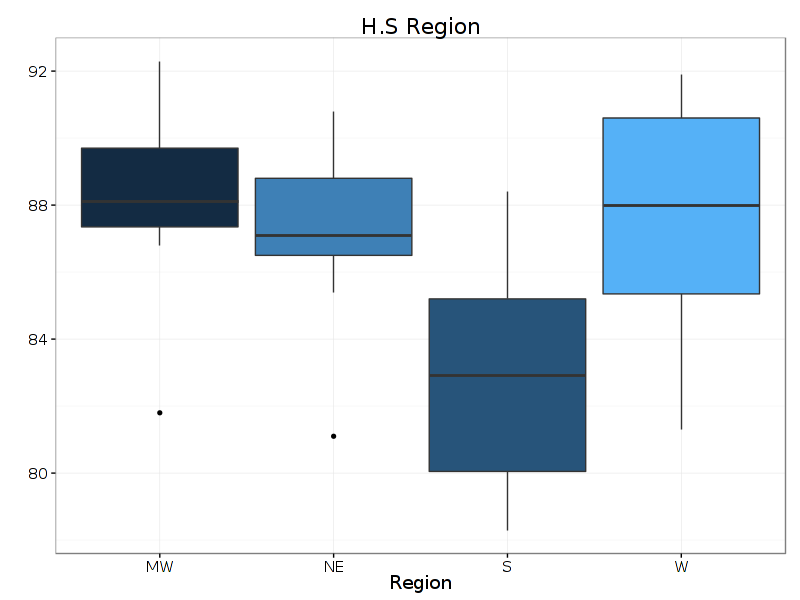H.S Region92888480MWwWNERegion

Question

The question is which box plot has the greatest variability and which box plot has the least variability?help_outlineImage TranscriptioncloseH.S Region 92 88 84 80 MW wW NE Region fullscreen
Step 1

Basics of a boxplot:

A boxplot is based on the 5-number summary: (Minimum, Q1, Median, Q3, Maximum); Q1 and Q3 are respectively the first and third quartiles.

The lowest* number on the vertical axis, till which the whisker reaches, represents the minimum; the highest* number, till the whisker reaches, represents the maximum.

Of the box, the line/boundary corresponding to the lowest value on the vertical axis, represents Q1; the boundary corresponding to the highest value represents Q3.

The line inside the box represents the median.

The boxplot gives quite a good picture about the central tendency (median), dispersion (range and inter-quartile range), and shape (comparative lengths of the whiskers and relative positions of the median with respect to Q1 and Q3) of the distribution of a dataset. Moreover, it also marks the outliers in the dataset.

*If there are outliers, then the whiskers may not reach all the way to the outliers, and these would be marked by a symbol, along the line of the whiskers. In that case, the lowest and highest outliers would represent the minimum and maximum values in the dataset, respectively.

Step 2

Description:

The inter-quartile range or IQR is: IQR = (Q3 – Q1).

It is a simple measure of dispersion or variability in the dataset, which provides the range within which, the middle-most 50% of the observations lie.

Although the IQR is not readily displayed on a boxplot, it can be calculated quite easily, simply by identifying Q1...

Want to see the full answer?

See Solution

Want to see this answer and more?

Our solutions are written by experts, many with advanced degrees, and available 24/7

See Solution
Tagged in

Statistics